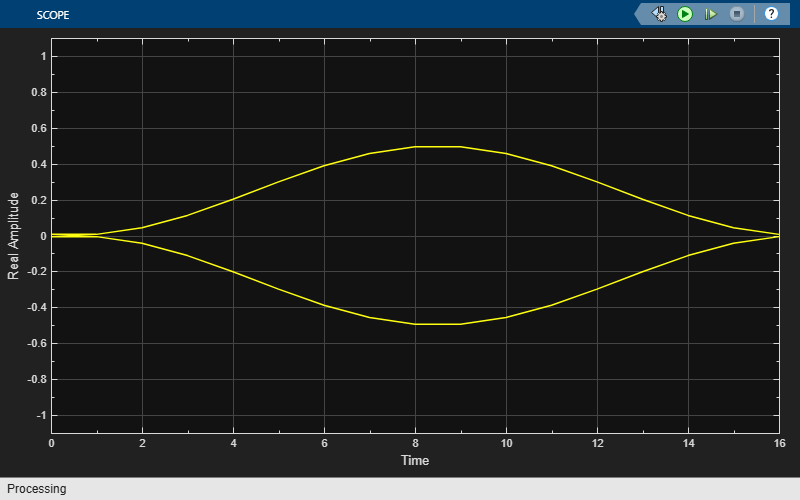# View CPM Phase Tree Using Simulink

This model uses the Eye Diagram block to view the in-phase and quadrature components, phase trajectory, phase tree, and instantaneous frequency of a CPM modulated signal.

### Explore Model

A random integer signal is converted to bits and then CPM modulated. The CPM modulated signal values are converted from complex to magnitude, and angle and then the phase is unwrapped. Eye diagram blocks are named to reflect the signal each displays.### Plot Eye Diagrams

When you run the example, several Eye Diagram blocks show how the CPM signal changes over time:

• The Modulated Signal block displays the in-phase and quadrature signals. Double-click the block to open the scope. The modulated signal is easy to see in the eye diagram only when the Modulation index parameter in the CPM Modulator Baseband block is set to 1/2. For a modulation index value of 2/3, the modulation is more complex and the features of the modulated signal are difficult to decipher. Unwrapping the phase and plotting it is another way to illustrate these more complex CPM modulated signals.

• The Phase Trajectory block displays the CPM phase. Double-click the block to open the scope. The Phase Trajectory block reveals that the signal phase is also difficult to view because it drifts with the data input to the modulator.

• The Phase Tree block displays the phase tree of the signal. The CPM phase is processed by a few simple blocks to make the CPM pulse shaping easier to view. This processing holds the phase at the beginning of the symbol interval and subtracts it from the signal. This resets the phase to zero every three symbols. The resulting plot shows the many phase trajectories that can be taken by the signal from any given symbol epoch.

• The Instantaneous Frequency block displays the instantaneous frequency of the signal. The CPM phase is differentiated to produce the frequency deviation of the signal. Viewing the CPM frequency signal enables you to observe the frequency deviation qualitatively, as well as make quantitative observations, such as measuring peak frequency deviation.

Running the model opens and plots the phase tree and instantaneous frequency eye diagram plots.### Exploring the Example

To learn more about the example, try changing the following parameters in the CPM Modulator Baseband block:

• Change Pulse length to a value between 1 and 6.

• Change Frequency pulse shape to one of the other settings, such as Rectangular or Gaussian.

You can observe the effect of changing these parameters on the phase tree and instantaneous frequency of the modulated signal.